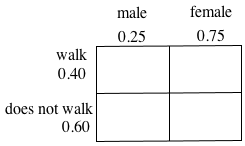### Home > GC > Chapter 6 > Lesson 6.2.4 > Problem6-74

6-74.

Assume that $25\%$ of the student body at your school is male and that $40\%$ of the students walk to school. If a student from this school is selected at random, find the following probabilities.

1. P(student is female)

2. P(student is male and does not walk to school)

3. P(student walks to school or does not walk to school)

Look at the percentage of the student body that is male.
If the total sum of females and males must be $100\%$, how much is left for females?

Make an area model.
Where on the table is P(male and does not walk)?

Where on the table is P(walk or does not walk)?